Support

Apps

•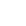My Wallet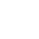My Order
•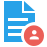My Profile
•My Connections
•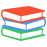My Books
•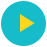My Videos
•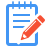My Tests
•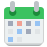My Calender
•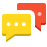My Messages
•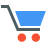My Shopping Cart
•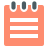My Orders
•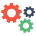Account Settings
•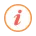Help

# Book Details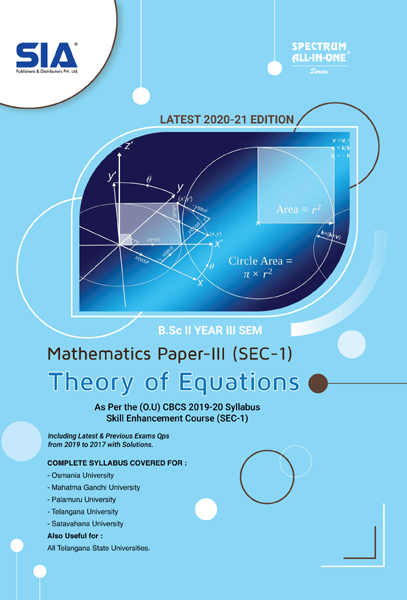# Theory of Equations (Mathematics Paper-III)

 Course Code : B.Sc III-Sem (O.U) ISBN : 9700112234035 Author : SIA PUBLISHERS University : Osmania University Regulation : 2021 Categories : Arts and Science Format :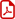PDF (DRM Protected) Type : eBook

Rs.99 Rs.55 Rs.45% off

Description :

SYLLABUS

Unit–I

General Properties of Polynomials Graphic representation of a polynomial – Maxima and minima values of polynomials – Theorems relating to the real roots of equations – Existence of a root in the general equation – Imaginary roots – Theorem determining the number of roots of an equation – Equal roots – Imaginary roots enter equations in pairs –Descartes’ rule of signs for positive roots – Descartes’ rule of signs for negative roots.

Unit–II

Roots and Coefficients of Equations Relations between the roots and coefficients – Theorem – Applications of the theorem – Depression of an equation when a relation exists between two of its roots – The cube roots of unity – Symmetric functions of the roots–examples.

Note : Theory of Equations (Mathematics Paper-III, SEC-1) B.Sc III-Sem (O.U) As per the CBCS 2019-20 Syllabus, Latest 2020-21 Edition

##### Publisher Detail:

Publisher Name: SIA Publishers and Distributors (P) Ltd.

Contact Email Id: msultan@siaedugroup.com